# Plotting rational numbers on a number line Online Quiz

Following quiz provides Multiple Choice Questions (MCQs) related to Plotting rational numbers on a number line. You will have to read all the given answers and click over the correct answer. If you are not sure about the answer then you can check the answer using Show Answer button. You can use Next Quiz button to check new set of questions in the quiz.### Explanation

Step 1:

$\frac{2}{3}$ (A) lies between 0 and 1; $1\frac{1}{3}$ (B) lies between 1 and 2

Step 2: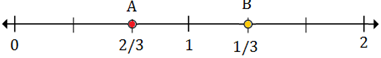### Explanation

Step 1:

$\frac{1}{4}$ (A) lies between 0 and 1; $1\frac{2}{4}$ (B) lies between 1 and 2

Step 2: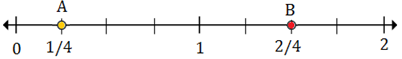### Explanation

Step 1:

$1\frac{2}{6}$ (A) lies between 1 and 2; $2\frac{5}{6}$ (B) lies between 2 and 3

Step 2:### Explanation

Step 1:

$-1\frac{4}{8}$ (A) lies between -1 and -2; $1\frac{5}{8}$ (B) lies between 1 and 2

Step 2: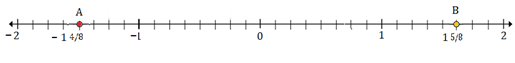### Explanation

Step 1:

$\frac{5}{8}$ (A) lies between 0 and 1; $2\frac{3}{8}$ (B) lies between 2 and 3

Step 2:### Explanation

Step 1:

$−\frac{1}{3}$ (A) lies between 0 and -1; $1\frac{2}{3}$ (B) lies between 1 and 2

Step 2: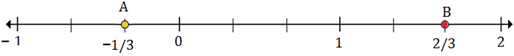### Explanation

Step 1:

$−\frac{2}{4}$ (A) lies between 0 and -1; $1\frac{3}{4}$ (B) lies between 1 and 2

Step 2:### Explanation

Step 1:

$−1\frac{3}{6}$ (A) lies between -1 and -2; $1\frac{4}{6}$ (B) lies btw 1 and 2

Step 2: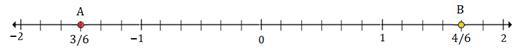### Explanation

Step 1:

$1\frac{1}{6}$ (A) lies between 1 and 2; $2\frac{3}{6}$ (B) lies between 2 and 3

Step 2: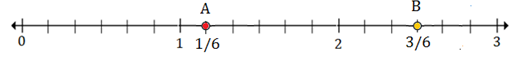### Explanation

Step 1:

$\frac{6}{8}$ (A) lies between 0 and 1; $2\frac{7}{8}$ (B) lies between 2 and 3

Step 2:plotting_rational_numbers_on_number_line.htm